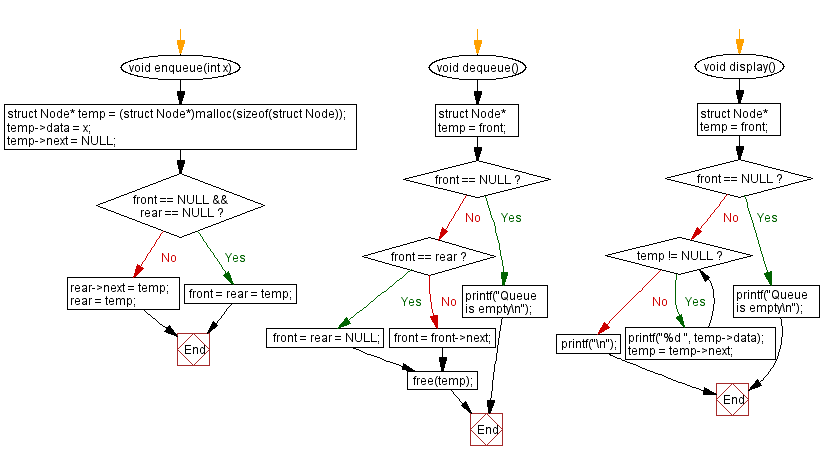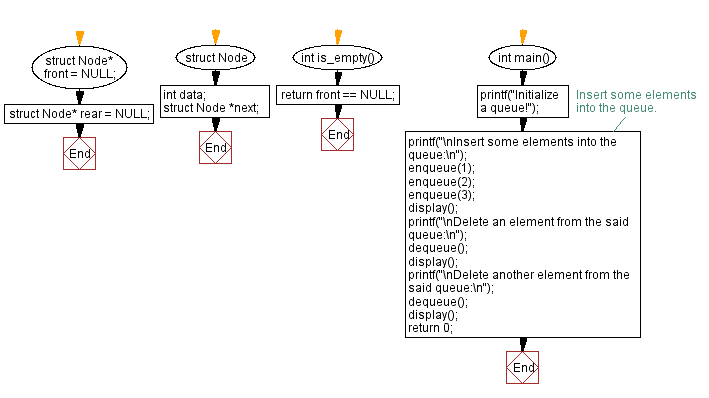﻿ C - Remove an element from a queue (using an linked list)

# C Exercises: Remove an element from a queue (using an linked list)

## C Queue: Exercise-4 with Solution

Write a C program to implement a queue using an array. Create a function that removes an element from the queue.

Sample Solution:

C Code:

``````#include <stdio.h>
#include <stdlib.h>

struct Node {
int data;
struct Node *next;
};

struct Node* front = NULL;
struct Node* rear = NULL;

void enqueue(int x) {
struct Node* temp =
(struct Node*)malloc(sizeof(struct Node));
temp->data = x;
temp->next = NULL;
if (front == NULL && rear == NULL) {
front = rear = temp;
return;
}
rear->next = temp;
rear = temp;
}

void dequeue() {
struct Node* temp = front;
if (front == NULL) {
printf("Queue is empty\n");
return;
}
if (front == rear) {
front = rear = NULL;
} else {
front = front->next;
}
free(temp);
}

void display() {
struct Node* temp = front;
if (front == NULL) {
printf("Queue is empty\n");
return;
}
while (temp != NULL) {
printf("%d ", temp->data);
temp = temp->next;
}
printf("\n");
}

int is_empty() {
return front == NULL;
}

int main() {
printf("Initialize a queue!");
// Insert some elements into the queue.
printf("\nInsert some elements into the queue:\n");
enqueue(1);
enqueue(2);
enqueue(3);
display();
printf("\nDelete an element from the said queue:\n");
dequeue();
display();
printf("\nDelete another element from the said queue:\n");
dequeue();
display();
return 0;
}
```
```

Sample Output:

```Initialize a queue!
Insert some elements into the queue:
1 2 3

Delete an element from the said queue:
2 3

Delete another element from the said queue:
3
```

Flowchart:C Programming Code Editor:

Have another way to solve this solution? Contribute your code (and comments) through Disqus.

What is the difficulty level of this exercise?

Test your Programming skills with w3resource's quiz.

﻿

## C Programming: Tips of the Day

C Programming - How do you pass a function as a parameter in C?

Declaration

A prototype for a function which takes a function parameter looks like the following:

```void func ( void (*f)(int) );
```

This states that the parameter f will be a pointer to a function which has a void return type and which takes a single int parameter. The following function (print) is an example of a function which could be passed to func as a parameter because it is the proper type:

```void print ( int x ) {
printf("%d\n", x);
}
```

Function Call

When calling a function with a function parameter, the value passed must be a pointer to a function. Use the function's name (without parentheses) for this:

```func(print);
```

would call func, passing the print function to it.

Function Body

As with any parameter, func can now use the parameter's name in the function body to access the value of the parameter. Let's say that func will apply the function it is passed to the numbers 0-4. Consider, first, what the loop would look like to call print directly:

```for ( int ctr = 0 ; ctr < 5 ; ctr++ ) {
print(ctr);
}
```

Since func's parameter declaration says that f is the name for a pointer to the desired function, we recall first that if f is a pointer then *f is the thing that f points to (i.e. the function print in this case). As a result, just replace every occurrence of print in the loop above with *f:

```void func ( void (*f)(int) ) {
for ( int ctr = 0 ; ctr < 5 ; ctr++ ) {
(*f)(ctr);
}
}
```

Ref : https://bit.ly/3skw9Um